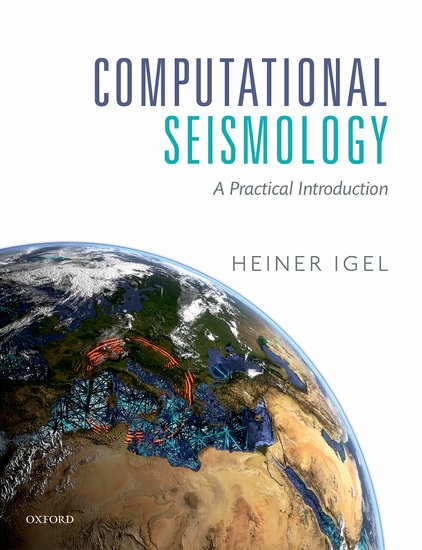{"cells": [{"cell_type": "markdown", "metadata": {}, "source": ["
\n", "
\n", "
\n", "
Computational Seismology
\n", "
Finite Differences - Advection Diffusion Reaction Equation
\n", "
\n", "
\n", "
"]}, {"cell_type": "markdown", "metadata": {}, "source": ["

\n", "\n", "\n", "\n", "

\n", "\n", "\n", "---\n", "\n", "This notebook is part of the supplementary material \n", "to [Computational Seismology: A Practical Introduction](https://global.oup.com/academic/product/computational-seismology-9780198717416?cc=de&lang=en&#), \n", "Oxford University Press, 2016.\n", "\n", "\n", "##### Authors:\n", "* Ashim Rijal ([@ashimrijal](https://github.com/ashimrijal))\n", "* Heiner Igel ([@heinerigel](https://github.com/heinerigel))"]}, {"cell_type": "markdown", "metadata": {}, "source": ["This exercise covers the following aspects:\n", "\n", "* Solving the advection-diffusion-reaction equation with different schemes of finite difference method\n", "* Investigation of different numerical solutions and finding the stable one\n", "---"]}, {"cell_type": "markdown", "metadata": {}, "source": ["## Basic Equations\n", "\n", "** Please refer to the Exercise 4.20 on Page 105 from the book.**\n", "\n", "The advection-diffusion-reaction equation is given by\n", "\n", "$$\n", "\\partial_t C(x,t) = k \\partial_x^2 C(x,t) + v \\partial_x C(x,t) - R(x)C(x,t) + P(x)\n", "$$\n", "Where,\n", "\n", "$C(x,t)$ is the concentration,\n", "\n", "$k$ is the diffusivity,\n", "\n", "$R(x)$ is the reactivity,\n", "\n", "$P(x)$ is the source, and\n", "\n", "$v$ is the advection velocity.\n", "\n", "We replace the derivatives as follows\n", "\n", "\n", "$$\n", "\\partial_x C(x,t) \\ \\approx \\ \\frac{C(x + \\mathrm{d}x, t) - C(x -\\mathrm{d}x,t)} {2 \\mathrm{d}x}\n", "$$\n", "\n", "$$\n", "\\partial_x^2 C(x,t) \\ \\approx \\ \\frac{C(x + \\mathrm{d}x, t) - 2C(x,t) + C(x - \\mathrm{d}x, t)}{\\mathrm{d}x^2}\n", "$$\n", "\n", "$$\n", "\\partial_t C(x,t) \\ \\approx \\ \\frac{C(x, t + \\mathrm{d}t) - C(x,t)}{ \\mathrm{d}t}\n", "$$\n", "\n", "The R.H.S. of the advection-diffusion-reaction becomes\n", "\n", "$$\n", "R.H.S. = dc = k \\bigg[ \\frac{C(x + \\mathrm{d}x) -2 C(x) + C(x - \\mathrm{d}x)}{\\mathrm{d} x^2} \\bigg] + v \\bigg[ \\frac{C(x + \\mathrm{d}x) - C(x -\\mathrm{d}x)}{2dx} \\bigg] - RC + P\n", "$$\n", "\n", "Now, solving for $C(x, t + \\mathrm{d}t)$ the extrapolation scheme is\n", "\n", "$$\n", "C(x, t + \\mathrm{d}t) = dc \\mathrm{d}t + C(t) = k \\mathrm{d}t \\bigg[ \\frac{C(x + \\mathrm{d}x) -2 C(x) + C(x - \\mathrm{d}x)}{\\mathrm{d} x^2} \\bigg] + v \\mathrm{d}t \\bigg[ \\frac{C(x + \\mathrm{d}x) - C(x -\\mathrm{d}x)}{2dx} \\bigg] - RC \\mathrm{d}t + P \\mathrm{d}t + C(t)\n", "$$\n", "\n", "**Note that we used centered finite-difference (in velocity term) scheme.**"]}, {"cell_type": "markdown", "metadata": {}, "source": ["### Exercise\n", "First understand the following codes, run the simulation and interpret the solution (plot). \n", "\n", "Then, implement the forward and the backward (in velocity term) finite-difference schemes. Investigate different numerical solutions. Which scheme is stable?\n", "\n", "**Message: Once you become familiar with all the codes below you can go to the Cell tab on the toolbar and click Run All.**\n"]}, {"cell_type": "code", "execution_count": null, "metadata": {"code_folding": , "lines_to_next_cell": 2}, "outputs": [], "source": ["# Configuration step (Please run it before the simulation code!)\n", "\n", "import numpy as np\n", "import matplotlib\n", "# Show Plot in The Notebook\n", "matplotlib.use(\"nbagg\")\n", "import matplotlib.pyplot as plt\n", "matplotlib.rcParams['figure.facecolor'] = 'w' # remove grey background\n", "from mpl_toolkits.mplot3d import Axes3D # for 3D plot"]}, {"cell_type": "code", "execution_count": null, "metadata": {"code_folding": [], "lines_to_next_cell": 2}, "outputs": [], "source": ["# Initialization of parameters\n", "\n", "k = 3*np.exp(4) # eddie mixing (diffusivity) in km^2/year\n", "v = 1500. # velocity km/year\n", "p0 = 0.01 # production rate in mol/year\n", "r = 2*np.exp(-3) # removal rate (reactivity) in mol/year\n", "\n", "# Choose among \"centered\", \"forward\" or \"backward\" finite-difference scheme.\n", "fd_type = \"forward\"\n", "#fd_type = \"centered\"\n", "#fd_type = \"backward\"\n", "\n", "# Space discretization\n", "nt = 300 # number of time steps\n", "nx = 100 # space dimension\n", "\n", "phi = 2*np.pi/(nx-1)*np.arange(0,nx) \n", "xc = np.cos(phi) # for visualization of eddie like circular plot\n", "yc = np.sin(phi) # for visualization of eddie like circular plot\n", "\n", "dt = 0.01 # years\n", "dx = 1000 # km\n", "isnap = 5 # snapshot frequency for visualization\n", "\n", "# Initialization of variables\n", "c = np.zeros(nx) # concentration\n", "dc =np.zeros(nx) # R.H.S. of equation\n", "p = np.zeros(nx) # source\n", "p[int((nx-1)/2)] = p0 # injecting source (production rate)"]}, {"cell_type": "code", "execution_count": null, "metadata": {"code_folding": [], "lines_to_next_cell": 0}, "outputs": [], "source": ["# Extrapolation scheme and the plots\n", "\n", "# Initialization of plot\n", "\n", "fig = plt.figure()\n", "ax = fig.gca(projection='3d')\n", "lines = ax.plot(xc,yc,c, 'r-', label = 'concentration')\n", "plt.legend(loc='best')\n", "plt.ion()\n", "plt.show()\n", "title = \"Concentration in\"\n", "\n", "# Begin extrapolation and update the plot\n", "# Circular shifting is done by using numpy function roll in the codes below\n", "\n", "for i in range(1,nt):\n", " if fd_type == \"centered\":\n", " \n", " dc = k * (np.roll(c,1) - 2 * c + np.roll(c,-1)) / (dx ** 2) + v * (np.roll(c,1) - \n", " np.roll(c,-1)) / (2 * dx) + p - r * c\n", "\n", " if fd_type == \"backward\":\n", " \n", " dc = k * (np.roll(c,1) - 2 * c + np.roll(c,-1)) / (dx ** 2) + v * (np.roll(c,0) - \n", " np.roll(c,-1)) / dx + p - r * c\n", " \n", " if fd_type == \"forward\":\n", " \n", " dc = k * (np.roll(c,1) - 2 * c + np.roll(c,-1)) / (dx ** 2) + v * (np.roll(c,1) - \n", " np.roll(c,0)) / dx + p - r * c\n", " \n", " \n", " # Time extrapolation scheme (Euler)\n", " cnew = c + dt * dc\n", " \n", " # The new presence is the current future!\n", " c = cnew\n", " \n", " c=c[nx-1] # Spatial boundary condition\n", "\n", " # Updating the plots\n", " if i % isnap == 0:\n", " for l in lines:\n", " l.remove()\n", " del l\n", " lines = ax.plot(xc,yc,c, 'r-')\n", " plt.title(title + \" time: %.2g years\" % (i * dt), loc = 'left')\n", " plt.gcf().canvas.draw()\n", " \n", "plt.ioff()\n", "plt.show() \n"]}, {"cell_type": "code", "execution_count": null, "metadata": {"lines_to_next_cell": 2}, "outputs": [], "source": []}], "metadata": {"kernelspec": {"display_name": "Python 3", "language": "python", "name": "python3"}}, "nbformat": 4, "nbformat_minor": 2}